# Foundations of Random Number Generation in JavaScript

### In this post, author Tim Brock goes over the basics of using random number generators in JavaScript, covering Math.random and a few simple (but still problematic) PNGs.

· Web Dev Zone · Analysis
Save
8.08K Views

being able to generate (apparently) random numbers is an essential requirement for many areas of math, statistics, the sciences, technology, and gaming. for instance, they can be used to assign participants to a group in a randomized controlled trial, to determine the output of a computer game, or to find approximate solutions to otherwise intractable problems. i frequently use random numbers to check my solutions to perfectly tractable statistical problems too. cryptographic random number generators, which we won't cover here, can be used in security.

## types of random number generator

for the purpose of this article, at least, we can think of there being three categories of random-number collection:

• "true" random numbers;
• pseudorandom numbers;
• quasirandom sequences.

true (or hardware) random number generators (trngs) use real-world physical processes believed to be random in nature to create streams of numbers. decay events from a radioactive source, for example, are random and uncorrelated with each other, atmospheric noise can also be used.

trngs often aren't practical or convenient (or necessary) for many purposes. a far more common alternative is to create a stream of numbers that appear to be randomly distributed over some interval using a computer algorithm. these algorithms are not truly unpredictable and so are referred to as pseudorandom number generators (prngs).

finally, quasirandom sequences are a finite collection of numbers that are meant to be representative of a sample space in some way. for example, the mean of the sequence may be the same (or very similar to) the known mean of the population. while quasirandom sequences are interesting and can be useful, they're not the focus of this article and won't be discussed further.

## the standard uniform distribution

generally, the output of a large number of values from a pseudorandom number generator is meant to approximate the standard uniform distribution (sud) that covers the range 0 to 1. there is, however, some variation in whether either/both of the end points are included. in the conceptual world of the mathematics of continuous distributions, there is basically no difference between the uniform distribution between [0,1] (includes 0 and 1) and the uniform distribution between (0,1) (excludes 0 and 1). in the real world of floating point numbers, with only a finite number of possible values between 0 and 1, the difference is real and could potentially be problematic. one might, for example, want to use the generated number inside the natural logarithm function math.log .

generating a random number for another distribution is usually "just" a matter of using one or more numbers from an sud prng to produce an appropriate value from the distribution of interest. depending on the desired final distribution, this might involve one line of code or something quite complex. for the rest of this article, i will stick to discussing the generation of numbers from a sud prng.

## period

a useful prng must have a large period, which is to say that it must be able to output a large number of numbers without repeating itself. for example, the wichmann hill prng of 1982 (more on this later) has a period of nearly 7 trillion numbers, while the exceedingly popular mersenne twister prng has a period of 2 19937 − 1 numbers. the former is considered quite short by modern standards.

## what's wrong with math.random ?

you may use javascript's built-in math.random regularly and have no problems with it. it does, however, have one big limitation: its output is not reproducible. run code that utilizes math.random again and again and you'll get a different set of results each time. in many cases, that really isn't a problem. in fact, in many (most, probably) cases it'll be exactly what you want. unpredictability (to a human sat staring at the output at least) is exactly what is required. but sometimes we do want to see the same set of results.

moving away from javascript for a moment, consider running a simulation experiment for a piece of work you wish to publish. perhaps you're using c++ or java or r or... you want your results to be reproducible. these languages (and many others besides) offer a way of "seeding" the initial state of their prngs. you set the seed in some way or other and you get the same sequence of "random" numbers out. math.random requires a seed too, there's just no way of setting it yourself. the specification for math.random is also fairly open-ended, allowing browser vendors to use "an implementation-dependent algorithm" so long as the output is approximately uniform over the range [0,1].

from a personal perspective, i'm a big fan of browser-based interactive data visualization for communicating both data and concepts. this could include simulation; there are limits to what is practical in a browser but web workers can help. simulation frequently requires random numbers. if the random numbers are not reproducible then the conditions for a simulation can't be re-run. there are plenty of other use cases too, such as a repeatable animation, and javascript isn't just the programming language of the browser anymore.

## problematic prngs

up until now, i've skipped over how uniform-distribution prngs work. it's all been something of a black box: you call a function one or more times, possibly setting a seed, and then some pseudorandom numbers come out. the problem is that creating a good prng is difficult. there are thousands of papers on the topic and multiple methods. and multiple instances where people who know much more about this topic than i appear to have got things wrong. for instance...

### randu

randu is a "linear congruential generator" (lcg) developed by ibm in the 1950's. lcg's use a recurrence relation of the following form to generate new pseudorandom numbers: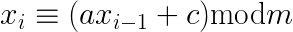in the case of randu, c is 0, a is 65,539, and m is 2 31 . because c is 0, randu is a member of a subset of lcg's called "multiplicative congruential generators" (mcg). to get a number in the range 0 to 1 (as is desired for a replacement for math.random ), one just needs to divide the result of the randu recurrence relation by m . a javascript implementation (which you definitely shouldn't use!) could look something like this:

``````var randu = function(seed){

"use strict";

if(!isfinite(seed)){
throw new error("seed not a finite number");
}

var x = math.round(seed);
var a = 65539;
var m = math.pow(2, 31);

if(x<1 || x≥m){
throw new error("seed out of bounds");
}

return function(){
x = (a*x)%m;
return x/m;
};

};
``````

the parity of the value generated by the recurrence relation in randu never changes. that is to say, an odd seed gives rise only to odd values of x while an even seed gives rise only to even values of x . this isn't exactly a desirable property, but there are bigger problems.

the period of an lcg is at most m , but for randu it is much less than that and depends on the parity of the seed. for odd seeds, it's over 536 million but for even seeds, it can be as little as 16,384 .

there's another reason not to bother with an even seed: one common, simple method for assessing the randomness of a generator is to plot pairs of successive values as a scatterplot. a good prng should fill a 1-by-1 square fairly evenly. with 10,000 random numbers, 5,000 points, and a seed of 1 everything looks reasonable. (you can think of "x" as referring to the values in even-index positions in an (0-indexed) array of 10,000 random numbers. that is indices 0, 2, 4, 6... 9,996, 9,998. points on the scatter plot are made by matching up with the next odd-index (1, 3, 5, 7... 9,997, 9999) "y" value.)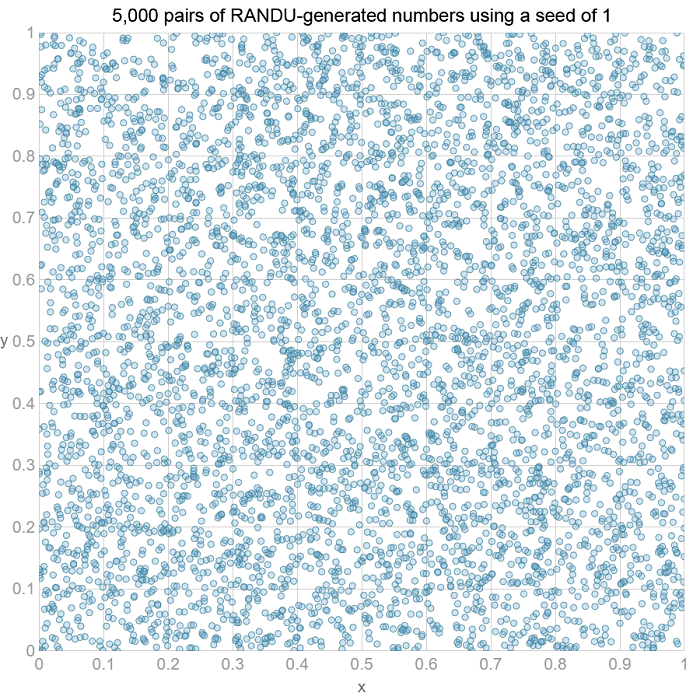with some even seeds we something quite different. below is a scatterplot for the seed 32,768.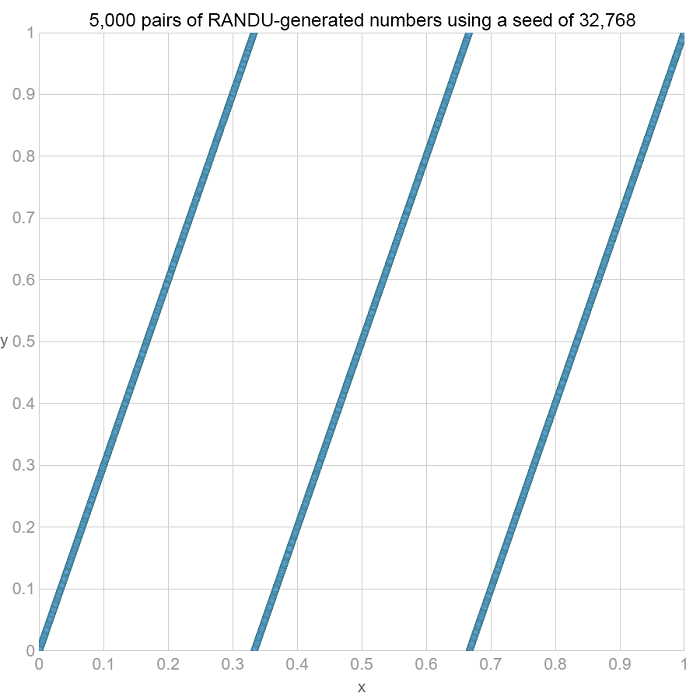clearly, we don't just have a deficit of points in the case of even seeds. in some cases, we have unambiguous relationships between neighboring values.

it would be simple enough to adapt the randu function above to reject even seeds, giving an appropriate error message. unfortunately, odds seeds show structure too. to see this, we just need to extend the scatterplot idea to three dimensions by looking at triplets of successive random numbers. 3d scatter plots are frequently pretty useless. randu data provides something of an exception to this rule. (here the indices for "x" values are 0, 3, 6, 9... 9,993, 9,996, the indices for "y" values are 1, 4, 7, 10... 9,994, 9997, and the indices for "z" values are 2, 5, 8, 11... 9,995, 9,998.)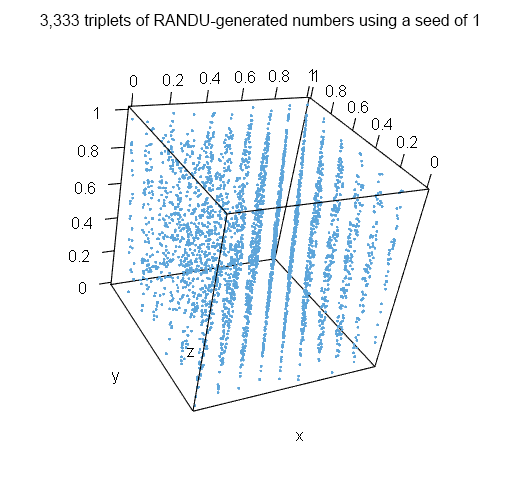rather than fill the box roughly evenly, triplets of numbers all lie in one of 15 planes, regardless of the seed! (actually, for the even seed 32,768 it's worse than this.) we can compare this with results from math.random (i used chrome for this); the difference is stark.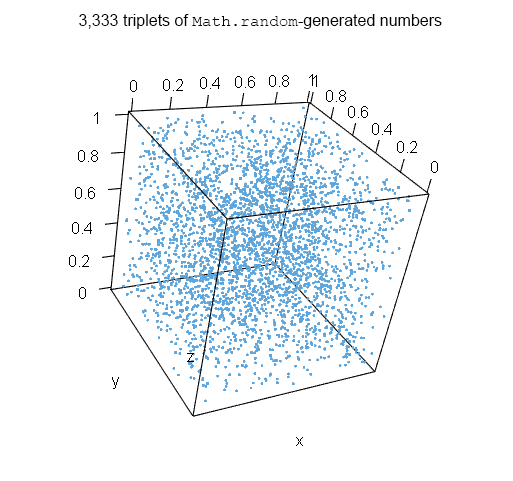one general problem with 3d scatterplots is that the visibility of structure in the plot can depend on the viewing angle. from certain angles, the structure in randu plot is hidden. this could be the case for math.random . to help with this issue, i created an interactive version that lets you choose a prng (and, where appropriate, one or more seeds) and visualizes the results using webgl . this demo can be found here and the box of random numbers can be rotated (using the mouse) and viewed from different angles. i've yet to find any obvious signs of structure when using math.random across a number of browsers (chrome, firefox, maxthon, ie, edge, and opera on the desktop and safari on ios).

the appearance of lattice structures in 3 or more dimensions exists for all mcg's, but it is particularly bad for randu. it has been known about since the 1960's . despite this, randu was still used in the 1970's and some simulation results from that era should, perhaps, be viewed with skepticism .

### excel

you may be wondering whether problems with prng's were confined to the 1960's and '70's? the short answer to this question is "no". after criticism of its old random number generator, microsoft changed to the wichmann–hill prng for excel 2003. the wh generator (first published in 1982 ) combines three mcg's to overcome some of the shortcomings of single mcgs (such as a relatively short period and the lattice planes and hyperplanes seen when looking at groups of neighboring values). a quick javascript implementation of wh could look like the following:

``````var wh = function(seeds){

"use strict";

if(seeds.length<3){
throw new error("not enough seeds");
}

var xa = math.round(seeds);
var aa = 171;
var ma = 30269;

var xb = math.round(seeds);
var ab = 172;
var mb = 30307;

var xc = math.round(seeds);
var ac = 170;
var mc = 30323;

if(!isfinite(xa) || !isfinite(xb) || !isfinite(xc)){
throw new error("seed not a finite number");
}

if(math.min(xa,xb,xc)<1 || xa≥ma || xb≥mb || xc≥mc){
throw new error("seed out of bounds");
}

return function(){
xa = (aa*xa)%ma;
xb = (ab*xb)%mb;
xc = (ac*xc)%mc;
return (xa/ma + xb/mb + xc/mc) % 1;
};

};

``````

again we can look for structure when plotting sets of neighboring values in two or three dimensions: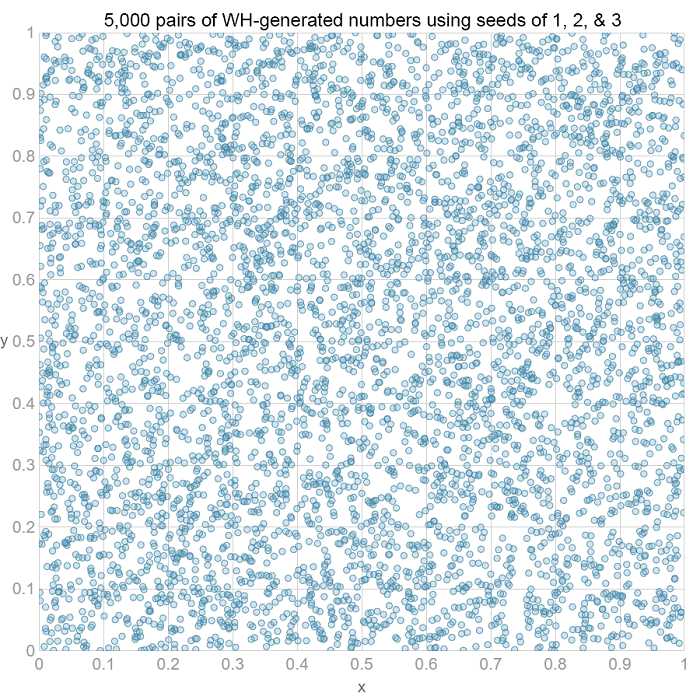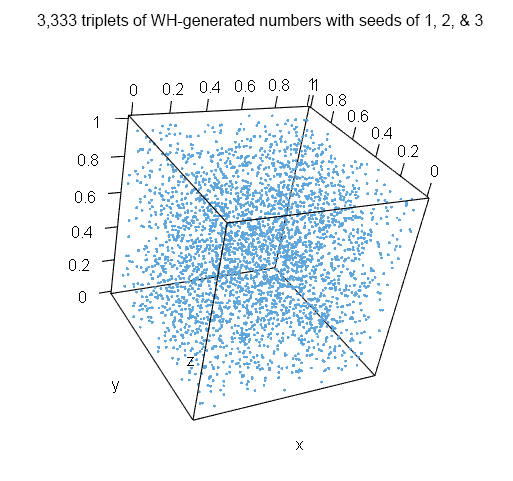while this clearly isn't sufficient to say whether or not we have a good random number generator, the plots above at least look reasonable. (you can also check the three-dimensional box in the webgl demo mentioned above.) however, the original implementation of the wh algorithm for the rand function in excel occasionally spat out negative numbers !

the wh algorithm fails a number of more modern and stringent tests of prngs and it seems microsoft has moved on to using the popular (but rather more complex) mersenne twister algorithm.

### v8

earlier i used output from chrome's implementation of math.random to illustrate what the distribution of triplets of random numbers should look like when plotted as a three-dimensional scatterplot. however, the algorithm that was used by v8, chrome's javascript engine, was recently shown to be flawed. specifically, it reproduced the same "random" character strings with far higher frequency than it should have, as this long but informative article describes. v8 developers promptly changed the algorithm used and the issue seems to have been fixed in chrome since version 49.

## speed

another consideration before you try to "grow your own" javascript prng is speed. one should expect math.random to be highly optimized. for example, some very rough tests using several browsers showed math.random to be ~3 times quicker at producing 100,000 random numbers than the simple wh implementation above. having said this, we're still talking about the order of just tens of milliseconds (at least in chrome and firefox on my laptop) so this may not be a bottleneck, even if you do require a large number of random numbers. of course, more complex prngs with better statistical properties may be slower.

## conclusions

i've barely touched the surface here. i've only looked at a couple of simple prngs and showed that they can still be problematic. there are far more complicated algorithms for prngs out there, some that have passed a large number of quite stringent statistical tests. and some of these already have open source javascript implementations.

if you don't need reproducibility, math.random may be just fine for all your random number needs. either way, if reliability of your random number generator is critical to how your site functions then you should perform relevant checks. in the case of math.random this means checking in all target browsers since the javascript specification does not specify a particular algorithm that vendors must use.

Topics:
random, number, large, generators, error

Published at DZone with permission of Tim Brock, DZone MVB.

Opinions expressed by DZone contributors are their own.